# MP Board Class 6th Maths Solutions Chapter 4 Basic Geometrical Ideas Ex 4.1

## MP Board Class 6th Maths Solutions Chapter 4 Basic Geometrical Ideas Ex 4.1

Question 1.
Use the figure to name :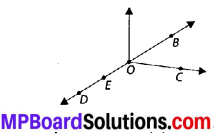(a) Five points
(b) A line
(c) Four rays
(d) Five line segments
Solution:
(a) Five points : O, B, C, D, E
(b) A line $$\overleftrightarrow { DB }$$
(c) Four rays $$\overrightarrow{O D}$$, $$\overrightarrow{O E}$$, $$\overrightarrow{O C}$$, $$\overrightarrow{O B}$$
(d) Five line segments :
$$\overline{D E}$$, $$\overline{O E}$$, $$\overline{O C }$$, $$\overline{O B}$$, $$\overline{O D}$$.Question 2.
Name the line given in all possible (twelve) ways, choosing only two letters at a time from the four given.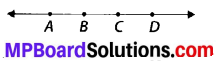Solution:
Possible lines are $$\overleftrightarrow { AB }$$, $$\overleftrightarrow { AC }$$, $$\overleftrightarrow { AD }$$, $$\overleftrightarrow { BC }$$, $$\overleftrightarrow { BD }$$, $$\overleftrightarrow { CD }$$, $$\overleftrightarrow { BA }$$, $$\overleftrightarrow { CA }$$, $$\overleftrightarrow { DA }$$, $$\overleftrightarrow { CB }$$, $$\overleftrightarrow { DB }$$, $$\overleftrightarrow { DC }$$.Question 3.
Use the figure to name :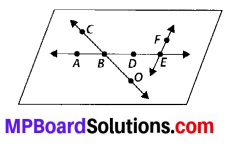(a) Line containing point E.
(b) Line passing through A.
(c) Line on which O lies
(d) Two pairs of intersecting lines.
Solution:
(a) A line containing point E is $$\overleftrightarrow { AE }$$.
(b) A line passing through A is $$\overleftrightarrow { AE }$$.
(c) A line on which O lies is $$\overleftrightarrow { CO }$$ or $$\overleftrightarrow { OC }$$.
(d) Two pairs of intersecting lines are $$\overleftrightarrow { AD }$$, $$\overleftrightarrow { CO }$$ and $$\overleftrightarrow { AE }$$, $$\overleftrightarrow { FE }$$.Question 4.
How many lines can pass through
(a) one given point?
(b) two given points?
Solution:
(a) Infinite number of lines can pass through one given point.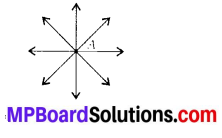(b) Only one line can pass through two given points.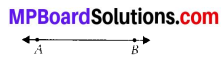Question 5.
Draw a rough figure and label suitably in each of the following cases:
(a) Point Plies on $$\overline{A B}$$.
(b) $$\overleftrightarrow { XY }$$ and $$\overleftrightarrow { PQ }$$ intersect at M.
(c) Line l contains E and F but not D.
(d) $$\overleftrightarrow { OP }$$ and $$\overleftrightarrow { OQ }$$ meet at O.
Solution: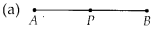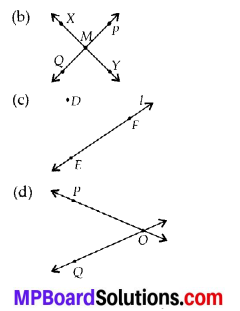Question 6.
Consider the following figure of line $$\overleftrightarrow { MN }$$. Say whether following statements are true or false in context of the given figure.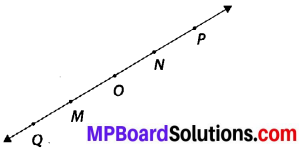(a) Q, M, O, N, P are points on the line $$\overleftrightarrow { MN }$$.
(b) M, O, N are points on a line segment $$\overline{M N}$$.
(c) M and N are end points of line segment $$\overline{M N}$$.
(d) O and N are end points of line segment $$\overline{O P}$$.
(e) M is one of the end points of line segment $$\overline{Q O}$$.
(f) M is point on ray $$\overrightarrow { OP }$$
(g) Ray $$\overrightarrow { OP }$$ is different from ray $$\overrightarrow { OP }$$.
(h) Ray OP is same as ray $$\overrightarrow { OM }$$.
(i) Ray $$\overrightarrow { OM }$$ is not opposite to ray $$\overrightarrow { OP }$$.
(j) O is not an initial point of $$\overrightarrow { OP }$$.
(k) N is the initial point of $$\overrightarrow { NP }$$ and $$\overrightarrow { NM }$$.
Solution:
(a) True
(b) True
(c) True
(d) False
(e) False
(f) False
(g) True
(h) False
(i) False
(j) False
(k) True